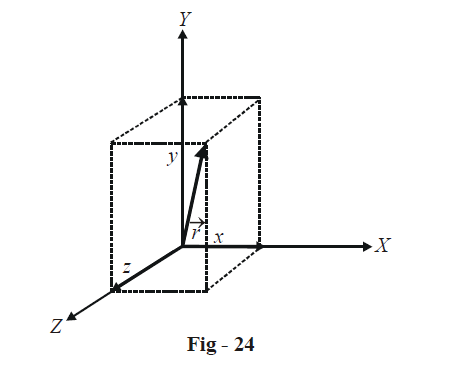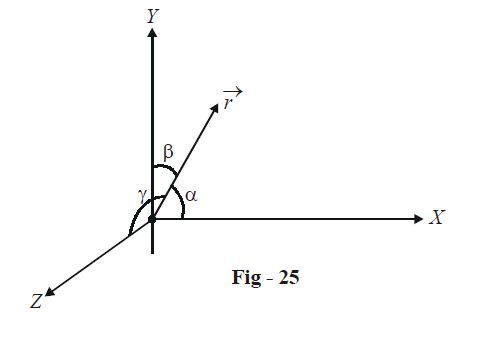# Magnitudes Direction Cosines And Direction Ratios Of Vectors

MAGNITUDE, DIRECTION COSINES AND DIRECTION RATIOS

Consider a vector

$\vec r = x\hat i + y\hat j + z\hat k$

as shown in the figure below:The magnitude or $$\vec r$$ is simply the length of the diagonal of the cuboid whose sides are x, y and z. Thus

$\left| {\vec r} \right| = \sqrt {{x^2} + {y^2} + {z^2}}\quad\quad\quad ...{\text{ }}\left( 1 \right)$

Suppose $$\vec r$$ makes angles $$\alpha ,\beta \,\,{\text{and}}\,\lambda$$ with the X, Y and Z axis, as shown:Then the quantities

\begin{align}&{l = {\text{ }}cos\,\alpha } \\ &{m = {\text{ }}cos\,\beta } \\ &{n = {\text{ }}cos\,\gamma } \end{align}

are called the direction cosines of $$\vec r$$ (abbreviated as DCs. The DCs uniquely determine the direction of the vector. Note that since

$\vec r = x\hat i + y\hat j + z\hat k$

we have

\begin{align}&\qquad\quad x = \left| {\vec r} \right|\cos \alpha = l\left| {\vec r} \right| \hfill \\& \qquad\quad y = \left| {\vec r} \right|\cos \beta = m\left| {\vec r} \right| \hfill \\&\qquad\quad z = \left| {\vec r} \right|\cos \gamma = n\left| {\vec r} \right| \hfill \\&\Rightarrow~ \quad {x^2} + {y^2} + {z^2} = \left( {{l^2} + {m^2} + {n^2}} \right){\left| {\vec r} \right|^2} \hfill \\ \end{align}

From (1), this gives

$\boxed{{l^2} + {m^2} + {n^2} = 1}$

We can also infer from this discussion that the unit vector $$\hat r$$ along $$\vec r$$ can be written as

\begin{align}&\hat r = \frac{{\vec r}}{{\left| {\vec r} \right|}} = \frac{{x\hat i + y\hat j + z\hat k}}{{\left| {\vec r} \right|}} \hfill \\&\;\;= l\hat i + y\hat j + z\hat k \hfill \\ \end{align}

Direction ratios (DRs) of a vector are simply three numbers, say a, b and c, which are proportional to the DCs, i.e

$\frac{l}{a} = \frac{m}{b} = \frac{n}{c}$

It follows that DRs are not uniqe (DCs obviously are)

From a set of DRs {a, b, c}, the DCs can easily be deduced:

$\frac{l}{a} = \frac{m}{b} = \frac{n}{c} = \frac{{\sqrt {{l^2} + {m^2} + {n^2}} }}{{\sqrt {{a^2} + {b^2} + {c^2}} }} = \frac{1}{{\sqrt {{a^2} + {b^2} + {c^2}} }}$

$\Rightarrow \quad l = \frac{a}{{\sqrt {{a^2} + {b^2} + {c^2}} }},m = \frac{b}{{\sqrt {{a^2} + {b^2} + {c^2}} }},n = \frac{c}{{\sqrt {{a^2} + {b^2} + {c^2}} }}$

Before we go on to solving examples involving the concepts we’ve seen till now, you are urged to once again go over the entire earlier discussion we’ve had, so that the “big picture” is clear in your mind.

Vectors
grade 11 | Questions Set 1
Vectors
Vectors
grade 11 | Questions Set 2
Vectors